NCERT Solutions for Class 10 Maths Chapter 9 Some Applications of Trigonometry Ex 9.1 are part of NCERT Solutions for Class 10 Maths. Here we have given NCERT Solutions for Class 10 Maths Chapter 9 Some Applications of Trigonometry Ex 9.1.

 Board CBSE Textbook NCERT Class Class 10 Subject Maths Chapter Chapter 9 Chapter Name Some Applications of Trigonometry Exercise Ex 9.1 Number of Questions Solved 16 Category NCERT Solutions

## NCERT Solutions for Class 10 Maths Chapter 9 Some Applications of Trigonometry Ex 9.1

Question 1.
A circus artist is climbing a 20 m long rope, which is tightly stretched and tied from the top of a vertical pole to the ground. Find the height of the pole, if the angle made by the rope with the ground level is 30° (see figure).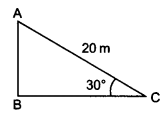Solution:
Given: length of the rope (AC) = 20 m, ∠ACB = 30°
Let height of the pole (AB) = h metres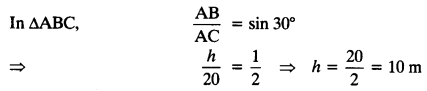Hence, height of the pole = 10 m

Question 2.
A tree breaks due to storm and the broken part bends so that the top of the tree touches the ground making an angle 30° with it. The distance between the foot of the tree to the point where the top touches the ground is 8 m. Find the height of the tree.
Solution:
Let DB is a tree and AD is the broken part of it which touches the ground at C.Question 3.
A contractor plans to install two slides for the children to play in a park. For the children below the age of 5 years, she prefers to have a slide whose top is at a height of 1.5 m, and is inclined at an angle of 30° to the ground, whereas for elder children, she wants to have a steep slide at a height of 3 m, and inclined at an angle of 60° to the ground. What should be the length of the slide in each case?
Solution:
Let l1 is the length of slide for children below the age of 5 years and l2 is the length of the slide for elder children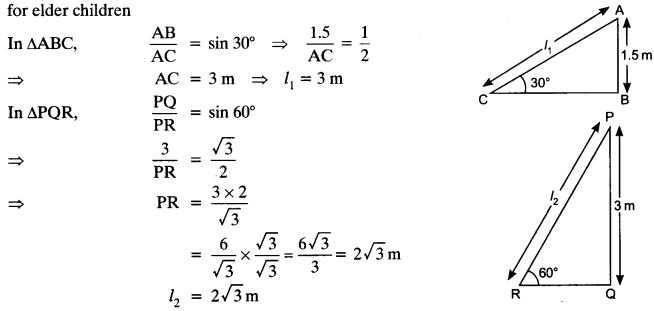Question 4.
The angle of elevation of the top of a tower from a point on the ground, which is 30 m away from the foot of the tower is 30°. Find the height of the tower.
Solution:
Let h be the height of the tower
In ∆ABC,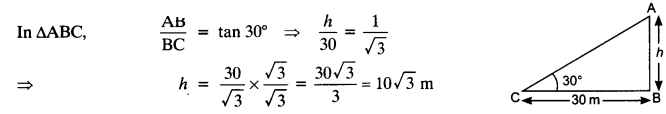Question 5.
A kite is flying at a height of 60 m above the ground. The string attached to the kite is temporarily tied to a point on the ground. The inclination of the string with the ground is 60°. Find the length of the string, assuming that there is no slack in the string.
Solution: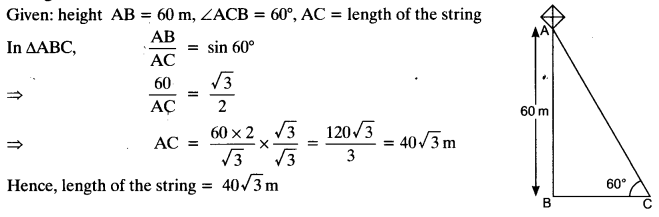Question 6.
A 1.5 m tall boy is standing at some distance from a 30 m tall building. The angle of elevation from his eyes to the top of the building increases from 30° to 60° as he walks towards the building. Find the distance he walked towards the building.
Solution:Question 7.
From a point on the ground, the angles of elevation of the bottom and the top of a transmission tower fixed at the top of a 20 m high building are 45° and 60° respectively. Find the height of the tower.
Solution: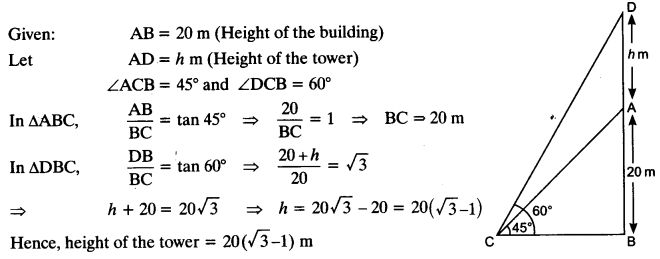Question 8.
A statue, 1.6 m tall, stands on the top of a pedestal. From a point on the ground, the angle of elevation of the top of the statue is 60° and from the same point the angle of elevation of the top of the pedestal is 45°. Find the height of the pedestal.
Solution:
Let the height of the pedestal AB = h m
Given: height of the statue = 1.6 m, ∠ACB = 45° and ∠DCB = 60°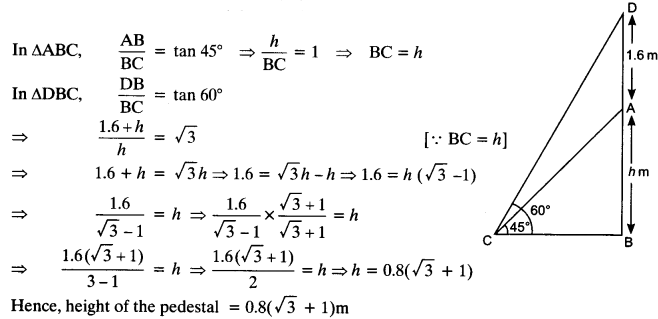Question 9.
The angle of elevation of the top of a building from the foot of a tower is 30° and the angle of elevation of the top of the tower from the foot of the building is 60°. If the tower is 50 m high, find the height of the building.
Solution:
Given: height of the tower AB = 50 m,
∠ACB = 60°, ∠DBC = 30°
Let the height of the building CD = x m
In ∆ABC,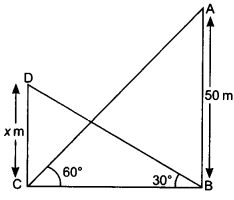Question 10.
Two poles of equal heights are standing opposite each other on either side of the road, which is 80 m wide. From a point between them on the road, the angles of elevation of the top of the poles are 60° and 30° respectively. Find the height of the poles and the distance of the point from the poles.
Solution:Question 11.
A TV tower stands vertically on a bank of a canal. From a point on the other bank directly opposite the tower, the angle of elevation of the top of the tower is 60°. From another point 20 m away from this point on the line joining this point to the foot of the tower, the angle of elevation of the top of the tower is 30° (see the given figure). Find the height of the tower and the width of the CD and 20 m from pole AB.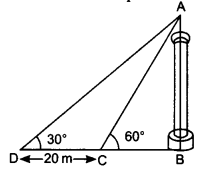Solution:
Let the height of the tower AB = h m and BC be the width of the canal.Question 12.
From the top of a 7 m high building, the angle of elevation of the top of a cable tower is 60° and the angle of depression of its foot is 45°. Determine the height of the tower.
Solution:
Let height of the tower AB = (h + 7) m
Given: CD = 7 m (height of the building),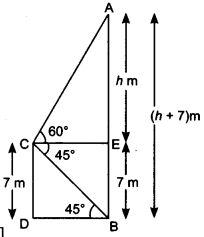Question 13.
As observed from the top of a 75 m high lighthouse from the sea-level, the angles of depression of two ships are 30° and 45°. If one ship is exactly behind the other on the same side of the lighthouse, find the distance between the two ships.
Solution:
Given: height of the lighthouse = 75 m
Let C and D are the positions of two ships.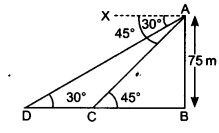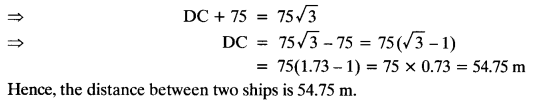Question 14.
A 1.2 m tall girl spots a balloon moving with the wind in a horizontal line at a height of 88.2 m from the ground. The angle of elevation of the balloon from the eyes of the girl at any instant is 60°. After sometime, the angle of elevation reduces to 30° (see figure). Find the distance travelled by the balloon during the interval.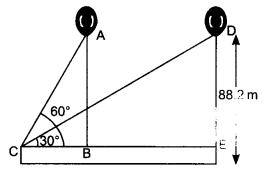Solution:
Let the first position of the balloon is A and after sometime it will reach to the point D.
The vertical height ED = AB = (88.2 – 1.2) m = 87 m.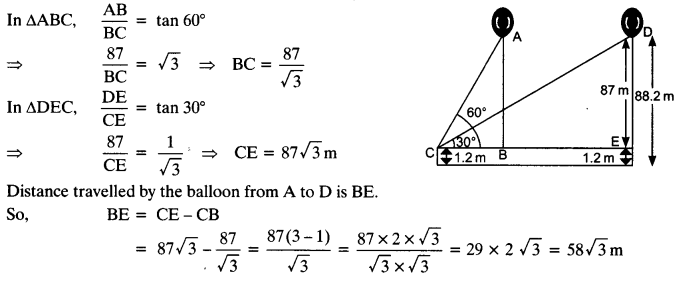Question 15.
A straight highway leads to the foot of a tower. A man standing at the top of the tower observes a car at an angle of depression of 30°, which is approaching the foot of the tower with a uniform speed. Six seconds later, the angle of depression of the car is found to be 60°. Find the time taken by the car to reach the foot of the tower from this point.
Solution:
Let the height of the tower AB = h m
and ∠XAC = ∠ACB = 60°
Let the speed of the car = x m/sec
Distance CD = 6 x x = 6x m
Let the time taken from C to B = t sec.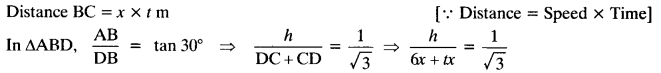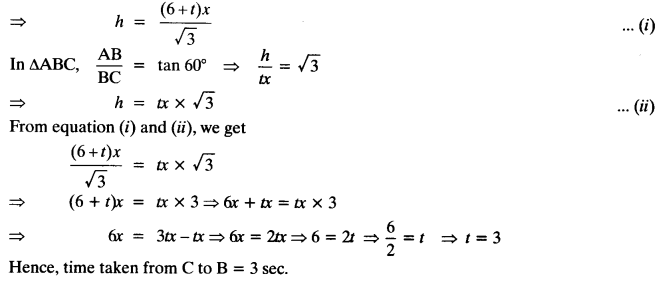Question 16.
The angles of elevation of the top of a tower from two points at a distance of 4 m and 9 m from the base of the tower and in the same straight line with it are complementary. Prove that the height of the tower is 6 m.
Solution:We hope the NCERT Solutions for Class 10 Maths Chapter 9 Some Applications of Trigonometry Ex 9.1 help you. If you have any query regarding NCERT Solutions for Class 10 Maths Chapter 9 Some Applications of Trigonometry Ex 9.1, drop a comment below and we will get back to you at the earliest.